Fredholm alternative

A statement of an alternative that follows from the Fredholm theorems. In the case of a linear Fredholm integral equation of the second kind,(1)

the Fredholm alternative states that either equation (1) and its conjugate equation(2)

have unique solutions, for any given functionsand, or the corresponding homogeneous equations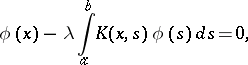(1prm)(2prm)

have non-zero solutions, where the number of linearly independent solutions is finite and is the same for both equations.

In the second case equation (1) has a solution if and only if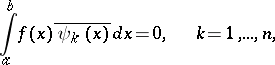whereis a complete system of linearly independent solutions of (2prm). Here the general solution of (1) has the formwhere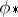is some solution of (1),is a complete system of linearly independent solutions of (1prm), and the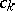are arbitrary constants. Similar statements also hold for equation (2).

Letbe a continuous linear operator mapping a Banach spaceinto itself; let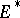and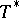be the corresponding dual space and dual operator. Consider the equations: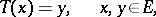(3)(4)(3prm)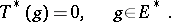(4prm)

The Fredholm alternative formeans the following: 1) either the equations (3) and (4) have solutions, for arbitrary right-hand sides, and then their solutions are unique; or 2) the homogeneous equations (3prm) and (4prm) have the same finite number of linearly independent solutionsand, respectively; in this case, for equation (3), or (4) respectively, to have a solution, it is necessary and sufficient that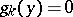,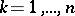, or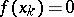,, respectively; here the general solution of (3) is given by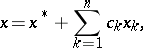and the general solution of (4) by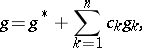where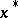(respectively,) is some solution of (3) ((4)), andare arbitrary constants.

Each of the following two conditions is necessary and sufficient for the Fredholm alternative to hold for the operator.

1)can be represented in the formwhereis an operator with a two-sided continuous inverse andis a compact operator.

2)can be represented in the formwhere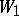is an operator with a two-sided continuous inverse andis a finite-dimensional operator.